# How to use Excel SUMIFS and SUMIF with multiple criteria

This tutorial explains the difference between the SUMIF and SUMIFS functions in terms of their syntax and usage, and provides a number of formula examples to sum values with multiple AND / OR criteria in Excel 365, 2021, 2019, 2016, 2013, 2010, and lower.

As everyone knows, Microsoft Excel provides an array of functions to perform various calculations with data. A few articles ago, we explored COUNTIF and COUNTIFS, which are designed for counting cells based on a single condition and several conditions, respectively. Last week we covered Excel SUMIF that adds values meeting the specified criteria. Now it's time to go over the plural version of SUMIF - Excel SUMIFS that allows summing values by multiple criteria.

Those who are familiar with the SUMIF function might think that converting it to SUMIFS takes just an extra "S" and a few additional criteria. This would seem quite logical… but "logical" it's not always the case when dealing with Microsoft : )

## Excel SUMIF function - syntax & usage

The SUMIF function is used to conditionally sum values based on a single criteria. We discussed the SUMIF syntax in detail in the previous article, and here's just a quick refresher.

SUMIF(range, criteria, [sum_range])
• range - the range of cells to be evaluated by your criteria, required.
• criteria - the condition that must be met, required.
• sum_range - the cells to sum if the condition is met, optional.

As you see, the syntax of the Excel SUMIF function allows for one condition only. And still, we say that Excel SUMIF can be used to sum values with multiple criteria. How can that be? By adding the results of several SUMIF functions and by using SUMIF formulas with array criteria, as demonstrated in the examples that follow.

## Excel SUMIFS function - syntax & usage

You use SUMIFS in Excel to find a conditional sum of values based on multiple criteria. The SUMIFS function was introduced in Excel 2007 and is available in all subsequent versions of Excel 2010, 2013, 2016, 2019, 2021, and Excel 365.

Compared to SUMIF, the SUMIFS syntax is a little bit more complex:

SUMIFS(sum_range, criteria_range1, criteria1, [criteria_range2, criteria2], …)

The first 3 arguments are mandatory, additional ranges and their associated criteria are optional.

• `sum_range` - one or more cells to sum, required. This can be a single cell, a range of cells or a named range. Only cells with numbers are summed; blank and text values are ignored.
• `criteria_range1` - the first range to be evaluated by the associated criteria, required.
• `criteria1` - the first condition that must be met, required. You can supply the criteria in the form of a number, logical expression, cell reference, text or another Excel function. For example you can use criteria such as 10, ">=10", A1, "cherries" or TODAY().
• `criteria_range2, criteria2, …` - these are additional ranges and criteria associated with them, optional. You can use up to 127 range/criteria pairs in SUMIFS formulas.

Notes:

• For a SUMIFS formula to work correctly, all the criteria_range arguments must have the same dimension as sum_range, i.e. the same number of rows and columns.
• The SUMIFS function works with AND logic, meaning that a cell in the sum range is summed only if it meets all of the specified criteria, i.e. all the criteria are true for that cell.

## Basic SUMIFS formula

And now, let's have a look at the Excel SUMIFS formula with two conditions. Suppose, you have a table listing the consignments of fruit from different suppliers. You have the fruit names in column A, suppliers' names in column B, and quantity in column C. What you want is to find out a sum of amounts relating to a given fruit and supplier, e.g. all apples supplied by Pete.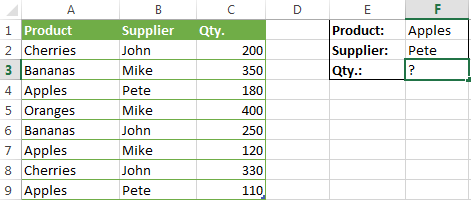When you're learning something new, it's always a good idea to start with simple things. So, to begin with, let's define all the arguments for our SUMIFS formula:

• sum_range - C2:C9
• criteria_range1 - A2:A9
• criteria1 - "apples"
• criteria_range2 - B2:B9
• criteria2 - "Pete"

Now assemble the above parameters, and you will get the following SUMIFS formula:

`=SUMIFS(C2:C9, A2:A9, "apples", B2:B9, "Pete")`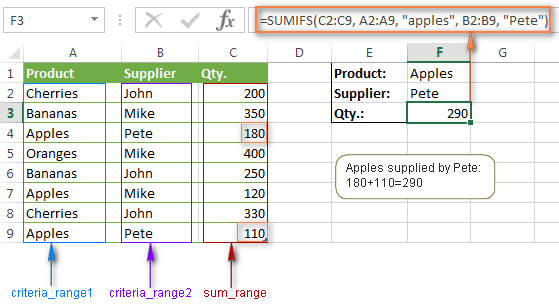To refine the formula further, you can replace the text criteria "apples" and "Pete" with cell references. In this case, you won't have to change the formula to calculate the quantity of other fruit from a different supplier:

`=SUMIFS(C2:C9, A2:A9, F1, B2:B9, F2)`

Note. Both the SUMIF and SUMIFS functions are case-insensitive by nature. To get them to recognize the text case, please see Case-sensitive SUMIF and SUMIFS formula in Excel.

## SUMIF vs. SUMIFS in Excel

Since the aim of this tutorial is to cover all possible ways to sum values by several conditions, we will discuss formula examples with both functions - Excel SUMIFS and SUMIF with multiple criteria. To use them correctly, you need to clearly understand what these two functions have in common and in what way they are different.

While the common part is clear (similar purpose and parameters), the differences are not so obvious, though very essential.

There are 4 major differences between SUMIF and SUMIFS:

1. Number of conditions. SUMIF can evaluate just one condition at a time while SUMIFS can check for multiple criteria.
2. Syntax. With SUMIF, the sum_range is the last and optional argument - if not defined, the values in the range argument are summed. With SUMIFS, sum_range is the first and required argument.
3. Size of ranges. In SUMIF formulas, sum_range does not necessarily have to be of the same size and shape as range, as long as you have the top left cell right. In Excel SUMIFS, each criteria_range must contain the same number of rows and columns as the sum_range argument.

For example, SUMIF(A2:A9,F1,C2:C18) will return the correct result because the leftmost cell in the sum_range argument (C2) is right. So, Excel will make the correction automatically and include as many columns and rows in sum_range as there are in range.

A SUMIFS formula with unequally sized ranges will return a #VALUE! error.

4. Availability. SUMIF is available in all Excel versions, from 365 through 2000. SUMIFS is available in Excel 2007 and higher.

Alright, enough strategy (i.e. theory), let's get into the tactics (i.e. formula examples : )

## How to use SUMIFS in Excel - formula examples

A moment ago, we discussed a simple SUMIFS formula with two text criteria. In the same manner, you can use Excel SUMIFS with multiple criteria expressed by numbers, dates, logical expressions, and other Excel functions.

### Example 1. Excel SUMIFS with comparison operators

In our fruit suppliers table, suppose, you want to sum all deliveries by Mike with Qty. 200 or more. To do this, you use the comparison operator "greater than or equal to" (>=) in criteria2 and get the following SUMIFS formula:

`=SUMIFS(C2:C9,B2:B9,"Mike",C2:C9,">=200")`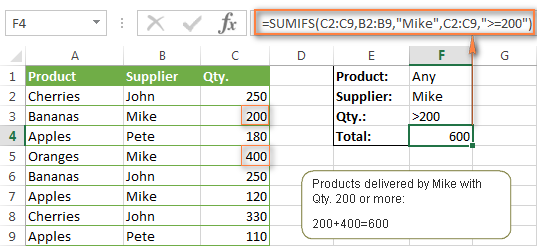Note. Please pay attention that in Excel SUMIFS formulas, logical expressions with comparison operators should always be enclosed in double quotes ("").

We covered all possible comparison operators in detail when discussing Excel SUMIF function, the same operators can be used in SUMIFS criteria. For example, the following formula with return the sum of all values in cells C2:C9 that are greater than or equal to 200 and less than or equal to 300.

`=SUMIFS(C2:C9, C2:C9,">=200", C2:C9,"<=300")`

### Example 2. Using Excel SUMIFS with dates

In case you want to sum values with multiple criteria based on the current date, use the TODAY() function in your SUMIFS criteria, as demonstrated below. The following formula sums values in column D if a corresponding date in column C falls within the last 7 days, including today:

`=SUMIFS(D2:D10, C2:C10,">="&TODAY()-7, C2:C10,"<="&TODAY())`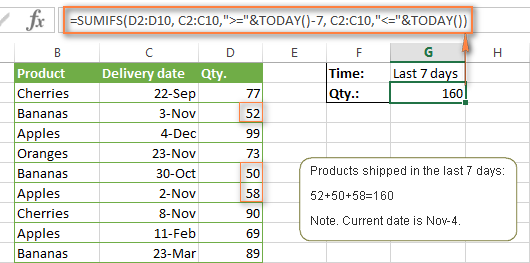Note. When you use another Excel function together with a logical operator in the criteria, you have to use the ampersand (&) to concatenate a string, for example "<="&TODAY().

In a similar fashion, you can use the Excel SUMIF function to sum values in a given date range. For example, the following SUMIFS formula adds the values in cells C2:C9 if a date in column B falls between 1-Oct-2014 and 31-Oct-2014, inclusive.

`=SUMIFS(C2:C9, B2:B9, ">=10/1/2014", B2:B9, "<=10/31/2014")`

The same result can be achieved by calculating the difference of two SUMIF functions, as demonstrated in this example - How to use SUMIF to sum values in a given date range. However, Excel SUMIFS is much easier and more understandable, isn't it?

### Example 3. Excel SUMIFS with blank and non-blank cells

When analyzing reports and other data, you may often need to sum values corresponding either to empty or non-empty cells.

Criteria Description Formula Example
Blank cells "=" Sum values corresponding to blank cells that contain absolutely nothing - no formula, no zero length string. =SUMIFS(C2:C10, A2:A10, "=", B2:B10, "=")

Sum values in cells C2:C10 if the corresponding cells in columns A and B are absolutely empty.

"" Sum values corresponding to "visually" blank cells including those that contain empty strings returned by some other Excel function (for example, cells with a formula like =""). =SUMIFS(C2:C10, A2:A10, "", B2:B10, "")

Sum values in cells C2:C10 with the same conditions as the above formula, but includes empty strings.

Non-blank cells "<>" Sum values corresponding to non-empty cells, including zero length strings. =SUMIFS(C2:C10, A2:A10, "<>", B2:B10, "<>")

Sum values in cells C2:C10 if the corresponding cells in columns A and B are not empty, including cells with empty strings.

SUM-SUMIF
or
SUM / LEN
Sum values corresponding to non-empty cells, not including zero length strings. =SUM(C2:C10) - SUMIFS(C2:C10, A2:A10, "", B2:B10, "")

=SUM((C2:C10) * (LEN(A2:A10)>0)*(LEN(B2:B10)>0))

Sum values in cells C2:C10 if the corresponding cells in columns A and B are not empty, cells with zero length strings are not included.

And now, let's see how you can use a SUMIFS formula with "blank" and "non-blank" criteria on real data.

Suppose, you have an order date in column B, delivery date in column C and Qty. in column D. How do you find the total of products that have not been delivered yet? That is, you want to know the sum of values corresponding to non-empty cells in column B and empty cells in column C.

The solution is to use the SUMIFS formula with 2 criteria:

`=SUMIFS(D2:D10, B2:B10,"<>", C2:C10,"=")`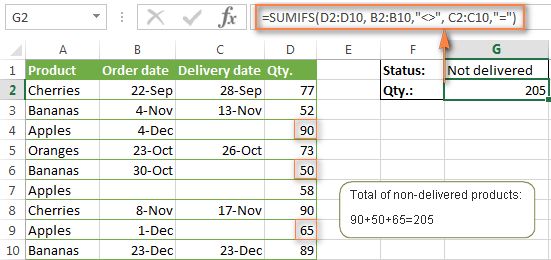## Using Excel SUMIF with multiple OR criteria

As noted in the beginning of this tutorial, the SUMIFS function is designed with AND logic. But what if you need to sum values with multiple OR criteria, i.e. when at least one of the conditions is met?

### Example 1. SUMIF + SUMIF

The simplest solution is to sum the results returned by several SUMIF functions. For example, the following formula demonstrates how to find the total of products delivered by Mike and John:

`=SUMIF(C2:C9,"Mike",D2:D9) + SUMIF(C2:C9,"John",D2:D9)`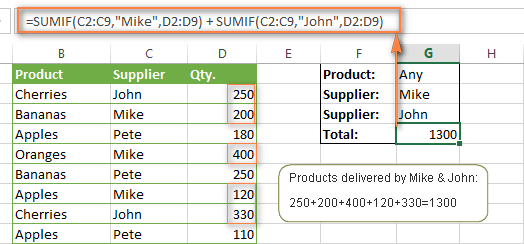As you see, the first SUMIF function adds the quantities corresponding to "Mike", the other SUMIF function returns the amounts relating to "John" and then you add these 2 numbers.

### Example 2. SUM & SUMIF with an array argument

The above solution is very simple and may get the job done quickly when there are only a couple of criteria. But a SUMIF + SUMIF formula may grow up enormously if you want to sum values with multiple OR conditions. In this case, a better approach is using an array criteria argument in the SUMIF function. Let's examine this approach now.

You can start by listing all of your conditions separated by commas and then enclose the resulting comma-separated list in {curly brackets}, which is technically called an array.

In the previous example, if you want to sum the products delivered by John, Mike and Pete, your array criteria will look like {"John","Mike","Pete"}. And the complete SUMIF function is `SUMIF(C2:C9, {"John","Mike","Pete"} ,D2:D9)`.

The array argument consisting of 3 values forces your SUMIF formula to return three separate results, but since we write the formula in a single cell, it would return the first result only - i.e. the total of products delivered by John. To get this array-criteria approach to work, you have to use one more little trick - enclose your SUMIF formula in a SUM function, like this:

`=SUM(SUMIF(C2:C9, {"John","Mike","Pete"} , D2:D9))`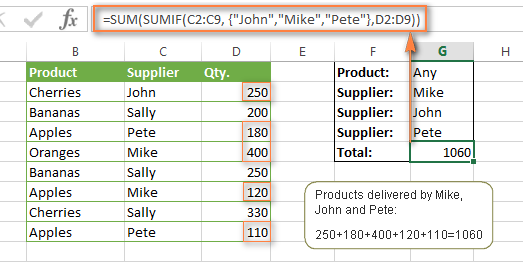As you see, an array criteria makes the formula much more compact compared to SUMIF + SUMIF, and lets you add as many values as you like in the array.

This approach works with numbers as well as with text values. For instance, if instead of the suppliers' names in column C, you had supplier IDs like 1, 2, 3 etc., then your SUMIF formula would look similar to this:

`=SUM(SUMIF(C2:C9, {1,2,3} , D2:D9))`

Unlike text values, numbers needn't be enclosed in double quotes in array arguments.

### Example 3. SUMPRODUCT & SUMIF

In case, your preferred way is to list the criteria in some cells rather that specify them directly in the formula, you can use SUMIF in conjunction with the SUMPRODUCT function that multiplies components in the given arrays, and returns the sum of those products.

`=SUMPRODUCT(SUMIF(C2:C9, G2:G4, D2:D9))`

Where G2:G4 are the cells containing your criteria, the suppliers' names in our case, as illustrated in the screenshot below.

But of course, nothing prevents you from listing the values in an array criteria of your SUMIF function if you want to:

`=SUMPRODUCT(SUMIF(C2:C9, {"Mike","John","Pete"}, D2:D9))`

The result returned by both formulas will be identical to what you see in the screenshot: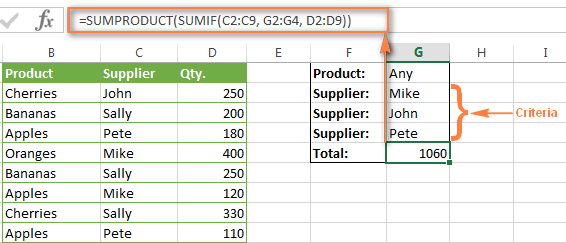## Excel SUMIFS with multiple OR criteria

If you want to conditionally sum values in Excel not simply with multiple OR conditions, but with several sets of conditions, you will have to use SUMIFS instead of SUMIF. The formulas will be very similar to what we've just discussed.

As usual, an example might help to illustrate the point better. In our table of fruit suppliers, let's add the Delivery Date (column E) and find the total quantity delivered by Mike, John and Pete in October.

### Example 1. SUMIFS + SUMIFS

The formula produced by this approach includes a lot of repetition and looks cumbersome, but it is easy to understand and, most importantly, it works : )

```=SUMIFS(D2:D9,C2:C9, "Mike", E2:E9,">=10/1/2014", E2:E9, "<=10/31/2014") + SUMIFS(D2:D9, C2:C9, "John", E2:E9, ">=10/1/2014", E2:E9, "<=10/31/2014") + SUMIFS(D2:D9, C2:C9, "Pete", E2:E9, ">=10/1/2014" ,E2:E9, "<=10/31/2014") ```

As you see, you write a separate SUMIFS function for each of the suppliers and include two conditions - equal to or greater than Oct-1 (">=10/1/2014",) and less than or equal to Oct 31 ("<=10/31/2014"), and then you sum the results.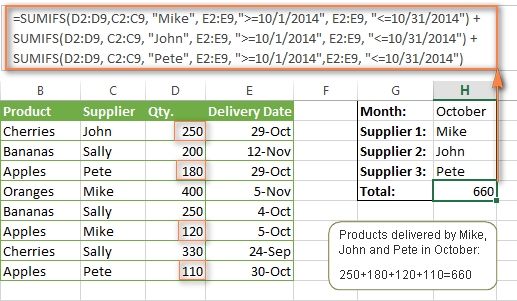### Example 2. SUM & SUMIFS with an array argument

I've tried to explain the essence of this approach in the SUMIF example, so now we can simply copy that formula, change the order of arguments (as you remember it is different in SUMIF and SUMIFS) and add additional criteria. The resulting formula is more compact than SUMIFS + SUMIFS:

`=SUM(SUMIFS(D2:D9,C2:C9, {"Mike", "John", "Pete"}, E2:E9,">=10/1/2014", E2:E9, "<=10/31/2014"))`

The result returned by this formula is exactly the same as you see in the screenshot above.

### Example 3. SUMPRODUCT & SUMIFS

As you remember, the SUMPRODUCT approach differs from the previous two in the way that you enter each of your criteria in a separate cell rather that specify them directly in the formula. In case of several criteria sets, the SUMPRODUCT function won't suffice and you will have to employ ISNUMBER and MATCH as well.

So, assuming that the Supplies Names are in cells H1:H3, Start Date is in cell H4 and End Date in cell H5, our SUMPRODUCT formula takes the following shape:

`=SUMPRODUCT(--(E2:E9>=H4), --(E2:E9<=H5), --(ISNUMBER(MATCH(C2:C9, H1:H3,0))), D2:D9)`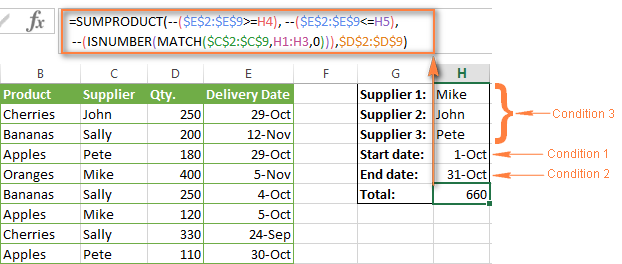Many people wonder why use double dash (--) in SUMPRODUCT formulas. The point is that Excel SUMPRODUCT ignores all but numeric values, while the comparison operators in our formula return Boolean values (TRUE / FALSE), which are non-numeric. To convert these Boolean values to 1's and 0's, you use the double minus sign, which is technically called the double unary operator. The first unary coerces TRUE/FALSE to -1/0, respectively. The second unary negates the values, i.e. reverses the sign, turning them into +1 and 0, which the SUMPRODUCT function can understand.

I hope the above explanation makes sense. And even if it doesn't, just remember this rule of thumb - use the double unary operator (--) when you are using comparison operators in your SUMPRODUCT formulas.

## Using Excel SUM in array formulas

As you remember, Microsoft implemented the SUMIFS function in Excel 2007. If someone still uses Excel 2003, 2000 or earlier, you will have to use a SUM array formula to add values with multiple AND criteria. Naturally, this approach works in modern versions of Excel 2013 - 2007 too, and can be deemed an old-fashioned counterpart of the SUMIFS function.

In the SUMIF formulas discussed above, you have already used array arguments, but an array formula is something different.

### Example 1. Sum with multiple AND criteria in Excel 2003 and earlier

Let's get back to the very first example where we found out a sum of amounts relating to a given fruit and supplier:As you already know, this task is easily accomplished using an ordinary SUMIFS formula:
`=SUMIFS(C2:C9, A2:A9, "apples", B2:B9, "Pete")`

And now, let's see how the same task can be fulfilled in early "SUMIFS-free" versions of Excel. First off, you write down all the conditions that should be met in the form of range="condition". In this example, we have two range/condition pairs:

Condition 1: A2:A9="apples"

Condition 2: B2:B9="Pete"

Then, you write a SUM formulas that "multiplies" all of your range/condition pairs, each enclosed in brackets. The last multiplier is the sum range, C2:C9 in our case:

`=SUM((A2:A9="apples") * ( B2:B9="Pete") * ( C2:C9))`

As illustrated in the screenshot below, the formula perfectly works in the latest Excel 2013 version.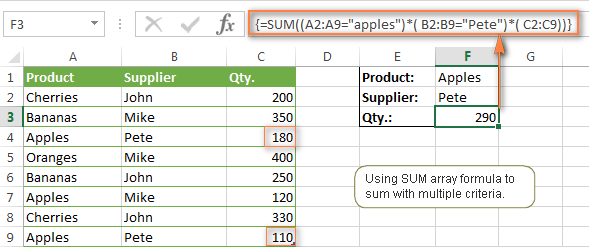Note. When entering any array formula, you must press Ctrl + Shift + Enter. Once you do this, your formula gets enclosed in {curly braces}, which is a visual indication that an array formula is entered correctly. If you try typing the braces manually, your formula will be converted to a text string, and it won't work.

### Example 2. SUM array formulas in modern Excel versions

Even in modern versions of Excel, the power of the SUM function should not be underestimated. The SUM array formula is not simply gymnastics of the mind, but has a practical value, as demonstrated in the following example.

Suppose, you have two columns, B and C, and you need to count how many times column C is greater than column B, when a value in column C is greater or equal to 10. An immediate solution that comes to mind is using the SUM array formula:

`=SUM((C1:C10>=10) * (C1:C10>B1:B10))`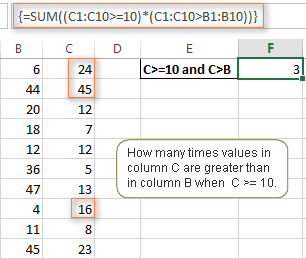Don't see any practical application to the above formula? Think about it in another way : )

Suppose, you have the orders list like shown in the screenshot below and you want to know how many products have not been delivered in full by a given date. Translated into Excel's language, we have the following conditions:

Condition 1: A value in column B (Ordered items) is greater than 0

Condition 2: A value in column C (Delivered) in less than in column B

Condition 3: A date in column D (Due date) is less than 11/1/2014.

Putting the three range/condition pairs together, you get the following formula:

`=SUM((B2:B10>=0)*(B2:B10>C2:C10)*(D2:D10<G2))`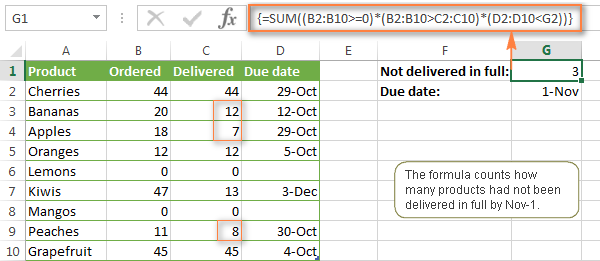Well, the formula examples discussed in this tutorial have only scratched the surface of what Excel SUMIFS and SUMIF functions can really do. But hopefully, they have helped pointing you in the right direction and now you can sum values in your Excel workbooks no matter how many intricate conditions you have to consider.

## You may also be interested in

1. Hi, I hope you could help.

I have a table where I need a sum to be displaying in the same cell, however it will vary but based on two options:

Sum for option 1 multiplied by the quantity
OR
Sum for option 2 multiplied by the quantity

• Hi!
The information you provided is not enough to understand your case and give you any advice.

2. Hi there

I want my cell to look for a set name (construction ref. A7) in another sheet and if that is true display the ammount of money spent so far but ONLY if its a positive number

Current construction has a loss of £500

I would like the loss to be shown in a second column by repeating the fomula but with 0)

but this is doing the maths of 900 minus -500 giving a return of 400

I dont know if i need to be combing VLOOKUP or if it should be a count etc? im very lost

• sorry the fomula got lost there

=SUMIF(Arnold_Sector,A7,Arnold_outcome)*(Arnold_outcome>0)

• Hello!
To display only a positive amount, use the formula:

=IF(SUMIF(B2:B9,A7,C2:C9)>0,SUMIF(B2:B9,A7,C2:C9),"")

To calculate the sum of only positive values by condition, use the formula:

=SUM((B2:B9=A7)*(C2:C9)*(C2:C9>0))

• Well i want it to comeplete the first task of looking to see if A7 is in the column, then look a the second column for the ammount related to it and add them up if theyre postive numbers

For example, i have got it to count the ammount of Constructions (2) and the total spent 1400 and i want it to look at the individual spends as a positive or negative and only add them up if theyre a positive (i can repeat the task for negative in a seperate cell)

thankyou for your help on this

3. Trying to figure out how to sum a row with date columns in it.
I want it to ignore negative numbers and the date.
Such as the following
"Buyer" "Amount" "Jan Date" "Amount" "Feb. Date" "Amount" "Mar. Date"............"Total Due"
Fred \$1000 1/1/21 -\$300 2/10/21 \$500 3/20/21
Sally \$300 1/20/21 -\$100 3/10/21

• Hello!
If I understand your task correctly, the following formula should work for you:

=SUM((A2:A5="Fred")*(B2:B5>0)*B2:B5,(A2:A5="Fred")*(D2:D5>0)*D2:D5)

If this is not what you wanted, please describe the problem in more detail.

4. Is there an easier way to type this sort of formula?

=SUMIFS(Sheet1!D:D;Sheet1!Q:Q;"TRUE";Sheet1!O:O;E16)+SUMIFS(Sheet1!E:E;Sheet1!Q:Q;"TRUE";Sheet1!O:O;E16)+SUMIFS(Sheet1!F:F;Sheet1!Q:Q;"TRUE";Sheet1!O:O;E16)+SUMIFS(Sheet1!G:G;Sheet1!Q:Q;"TRUE";Sheet1!O:O;E16)+SUMIFS(Sheet1!H:H;Sheet1!Q:Q;"TRUE";Sheet1!O:O;E16)

...and goes on like this for a few more columns. Using Excel 2016.
Thanks

• Hi!
It is very difficult to understand a formula that contains unique references to your workbook worksheets. Explain what you want to do.

5. Good evening sir, i have some questions as below:
1. Why need toput sum infront of sumif/sumifs? Any the result place sum(sumif/sumifs) is correct?
2. is there any easy way to get value number from the value text? Exp: i wanna get 100 in number from the text \$100.00?
thanks

6. I have created a weekly revenue record of a hospital spread over a month. I created it such that each sheet contains 3 month record by-weekly. Now my sheet 2 is supposed to be a summary sheet categorizing the income into the various hospital unit . I have used the SUMIFS Formula, it worked but could not place the revenue in its appropriate category.e.g revenue from pharmacy should go to pharmacy in the summary sheet while Revenue from laboratory should go to laboratory.but it is not so with what I created.I believe something is not right with my formula. What could be the problem and please can you help me out?
Thanks

• Hello!
Unfortunately, without seeing your data it is difficult to give you any advice. I recommend paying attention to the SUMIFS function. You can find the examples and detailed instructions in the article above.

7. hai thanks for this page, i can build 2 criteria and with 3 criteria.

with 2 criteria
=SUMIFS(\$G\$18:\$G\$501;\$D\$18:\$D\$501;"Apple";\$F\$18:\$F\$501;"23/06/2021")

with 3 criteria
=SUMIFS(\$G\$18:\$G\$501;\$D\$18:\$D\$501;"Apple";\$F\$18:\$F\$501;"23/06/2021";\$B\$18:\$B\$501;"city")

8. in sumif how we can get average no.

9. Thank you for these great explanations and clear examples. They helped me automate some manual things I have done over the past few years, and will help me reduce errors. Thanks!

10. Thank you for the formula and explanation of SUMIF(range;{array};range) to create an array of results and SUM(SUMIF(range;{array};range)) to sum the array of results into one cell! It helped me very much.

11. Hi, The formula that I am using is =SUMIF('FA Journal'!A1:A10000,L51,'FA Journal'!J6:J615). I need this formula for over 5000 rows when I drag the formula down, the range (A1:10000 becomes A2:A10001, I want criteria to keep change as per the row number but not the range. How can I resolve this issue? Thanks

• You can use A:A for range, which helps a bunch while using sumif and suimfs functions

12. I have a question about sumifs with multiple criteria. There is an example that shows products (orange, apple, banana), suppliers (Pete, Mike, ) and qty. I apply the similar logic/formula to a spreadsheet with a slightly different layout and it returns an error (#value). Imagine a spreadsheet where the name of the suppliers (Pete, Mike, etc.) are in row 1 and repeated across several columns multiple times. Now imagine in column A are the names of products (orange, banana, etc.) and they also appear in several rows multiple times. Inside the spreadsheet, where supplier/product intersects are the numbers (Qty). When I apply the same formula/logic), it returns error. My table has 10 columns (B to K) and 10 rows (A2 to A11). Here's my formula: =SUMIFS(B2:K11,B1:K1,"Pete",A2:A11,"apple"). This gives an error message (#value). Why wouldn't it count/add the quantities in the cells based on the criteria in this type of spreadsheet? I think it has something do with the table layout but can't figure it out.

• Hello!
The SUMIFS function works with conditions in only one column, not a range of data. Therefore, in your case, this function cannot be used.
Try this formula:

=SUMPRODUCT(B2:M9*(A2:A9=N1)*(B1:M1=P1))
N1 - product (apple)
P1 - supplier (Pete)

You can change it for your data.

• Hi Alexander, thank you but it is not working. I believe B2:M9 is the array (range) for the table/area that contains the values so that's what I selected. N1, P1, I used the values as +"apple", "pete" or was I supposed to create a separate tables to define P1 and N1? In any case, the formula resulted in error. Maybe you can test it at your end and send me an updated formula.

• Hello!
I wrote this formula based on the description you provided in your original comment. B2:M9 is the range that numbers (Qty) are in. N1 says "apple", P1 says "Pete". Column A says orange, apple, banana, apple, banana, etc. Row 1 says Pete, Mike, Pete, etc.
What doesn't work for you?

13. how to use for sumifs function for two tables example i have table 1 and table 2 have same structure with different data

TABLE 1 TABLE 2
TEAM 1 Credit TEAM 2 Credit
Cameron Bancroft 8.5 Josh Philippe(w) 8.5
Liam Livingstone 9 James Vince 9
Mitchell Marsh 10 Daniel Hughes 10
Colin Munro 8 Moises Henriques(c) 8
Josh Inglis(w) 9 Jordan Silk 9
Ashton Turner(c) 9 Daniel Christian 9
Aaron Hardie 9 Carlos Brathwaite 9
Jhye Richardson 10 Sean Abbott 10
Andrew Tye 8 Ben Dwarshuis 8
Jason Behrendorff 8 Steve OKeefe 8
Fawad Ahmed 10 Jackson Bird 10

i need output

OUTPUT TO BE LIKE THIS
PLAYING TEAM Credit
Daniel Hughes 10
Moises Henriques(c) 8
Jordan Silk 9
Cameron Bancroft 8.5
Liam Livingstone 9
Mitchell Marsh 10
Colin Munro 8
Josh Inglis(w) 9

• Hello!
If you need to apply the SUMIFS function to two tables, use the OR logic as shown in this tutorial.

14. Hi Alexander,

I need your help with summarizing my data.
I have data about 162 countries from 1970 to 2013. In each year, I have data about the frequency of s specific ethnic group. There are different ethnic groups for each country, and I'm trying to summarize for each country the data of all the frequencies in a given decade. I can't just roll the command of "sum" to all countries because they are all different in the number of ethnic group cells.
What can I do?

It looks like this:
country year ethnic group freq SUM
US 1970 muslim number SUMMARY for 1970
US 1970 jewish number
US 1970 romancatholic number
US 1970 non.reli number
US 1970 orthodox number

• Hello!
Here is the article that may be helpful to you: Vlookup multiple matches in Excel with one or more criteria.
Please describe your problem in more detail. Include an example of the source data and the result you want to get. It’ll help me understand your request better and find a solution for you.

15. great blog post

16. Hi Alexander,

I need help with a 2D sumifs function... My firm has had employees tract their time details in a single spreadsheet.... Column A is the start time (standard dates in 6 minute increments starting from "8:00:00 AM"), column B is the stop time, column c is the project on 1/1, column d is comments on 1/1, column e is the project on 2/2, column f is 2/2 comments etc.

I would like to write a single formula in a summary sheet where I can reference each employees timesheet, and list each project in column a and each date in row 1, and summarize the data in the resulting table. his would be a simple copy and paste formula if the comments weren't there, but i don't want to delete all those extra columns for every employee. I have seen articles with a 2D sumif, but the index(match() is always used on the sum and never on the criteria.

Thanks.

17. how can apply and (&) statement ( to sum different cells with multiple condition in SUMIFS function

• Hi,
The syntax for the SUMIF function does not include &. If you explain your problem in more detail, I will try to help you.

18. Hello,
I have multiple columns I need to be able to sort Month by Month and then I have 9 columns that I need to find a % of based off the date or the month.
Ex: DOB, Male, Female, in a relationship, single, bald, has hair, Left handed, right handed. What % of males are single, bald and right handed.
Any help would be greatly appreciated!

• Hello!
To count the number of specific values in a data range (for example, single, bald and right handed), use the COUNTIFS function

19. Hello there,

=SUMIF(C2:C9,"Mike",D2:D9) + SUMIF(C2:C9,"John",D2:D9)

this sums regardless of any product, what if i want it to sum based on the product without declaring it in the formula

Ex: get me the sum of Mike and Pete if the prodcuts were a match
it gets me the oranges sum alone and the apples alone without me specifying it in the formula

• Hello!
I recommend using the SUMIFS function, which calculates the amount according to several conditions. Please read the above article carefully.

20. I want to add sumifs 12 times( for calculation for 12 months). 3 additions are resulting correctly after that results are not as desired

• Hello!
I’m sorry but your task is not entirely clear to me. For me to be able to help you better, please describe your task in more detail. Please specify what you were trying to find, what formula you used and what problem or error occurred. Give an example of the source data and the expected result.
It’ll help me understand it better and find a solution for you

21. I want to add cell starting with 2 common letters. How can this be added to the formula.
Like
FL20.3 -- 40
FL30.1 --100
BL12.4 -- 10
TC10.001 -- 5
I want to add all FL20.3 and FL30.1. Is it possible?

• Hello!
I’m sorry but your task is not entirely clear to me. Could you please describe it in more detail? What result do you want to get? Thank you!

22. I am trying to create a SUMIF formula for a variety of fields.
This is my current formula
=SUMIFS('General Acct - 2020 Debits & Credits'!\$G\$581:\$G\$648,'General Acct - 2020 Debits & Credits'!\$B\$581:\$B\$648,{"Transfer to Secured Acct (Special Projects)","Transfer to Reserve Acct","Transfer to Hydro Acct"},'General Acct - 2020 Debits & Credits'!\$C\$581:\$C\$648,"Other")

The just of it is that if any of the "Transfer Accts" are chosen in column B and "Other" is chosen in Column C then then sum all amounts associated in column G (debit column)

right now if just returns 1 individual amount

• Hello Erika!
If I understand your task correctly, you can use the SUM function:

=SUM(SUMIFS(‘General Acct – 2020 Debits & Credits’!\$G\$581:\$G\$648,’General Acct – 2020 Debits & Credits’!\$B\$581:\$B\$648,{“Transfer to Secured Acct (Special Projects)”,”Transfer to Reserve Acct”,”Transfer to Hydro Acct”},’General Acct – 2020 Debits & Credits’!\$C\$581:\$C\$648,”Other”))

23. hi , iam traying to sum the time for user level in multiform time , date and user id
below is the example
PTW_USER_ID PTW_DATE PTW_TIME
58169 24-Jul-20 7:25:51
155129 24-Jul-20 7:28:45
58169 24-Jul-20 7:16:08
30124 24-Jul-20 7:18:04
30124 24-Jul-20 7:47:46
58169 24-Jul-20 7:12:10
111885 24-Jul-20 6:20:19
111885 25-Jul-20 0:05:55
111885 25-Jul-20 0:07:16
111730 25-Jul-20 0:44:54
111885 25-Jul-20 0:05:55
111885 25-Jul-20 0:07:16
111730 25-Jul-20 0:44:54
111020 26-Jul-20 0:01:57
111885 26-Jul-20 0:01:05
111020 26-Jul-20 0:28:10

• Hello!
I’m sorry but your task is not entirely clear to me. Could you please describe it in more detail? What result do you want to get?

24. I'm trying to come up with a way to calculate total time, by code, by date.
Example: how much time was coded, on the 21st for the K90 code?
Date Code Time
7/19/2020 NU0 4.18
7/21/2020 K90 5.33
7/21/2020 K90 0.5
7/21/2020 E42 2.67
7/20/2020 E42 4.92
7/20/2020 K90 8.48
Thank you for any help

• Hello Mike!
I hope you have studied the recommendations in the above tutorial.
If I understand your task correctly, the following formula should work for you:

=SUMIFS(D50:D55,B50:B55,DATE(2020,7,21),C50:C55,"K90")

25. As a general comment on SUMIFS; note that if none of the criteria are met, the result is zero, not an error of some kind.

27. sir,
I am using sumif formula to sum 3 columns data, but it giving 1column summary.
syntax is:SUMIF(G3:G619,H621,C3:E619).
pl advice , how to correct it.

I have a formula to sum values "TimeSheet!E:E" according to month selected "AC2" and by ID & Emp, but what I have been struggling with is the other part of the formula if a month is not selected and a quarter is selected.
Instead of months as text, I use 1-12 to simplify formula. This is the formula that I am using.

=SUM((MONTH(TimeSheet!\$G:\$G)=\$AC\$2)*(TimeSheet!\$B:\$B=\$B5)*(TimeSheet!\$J:\$J=E\$2)**(TimeSheet!\$E:\$E))

AC2 (Month 1-12/1Qtr, 2Qtr, 3Qtr, 4Qtr) B:B (Emp Column) E:E (Miles Column) G:G (Dates Column) J:J (ID Column)

The formula I have been trying is:

SUM(SUMIF(AC2,{"1","2","3","4","5","6","7","8","9","10","11","12"},TimeSheet!\$J:\$J=C\$2,TimeSheet!\$B:\$B=\$B5,(MONTH(TimeSheet!\$G:\$G)=\$AC\$2,TimeSheet!\$E:\$E),SUMPRODUCT((ROUNDUP(MONTH(TimeSheet!\$G:\$G)/3,0)=AC2,YEAR(TimeSheet!\$G:\$G)=AC2,TimeSheet!\$J:\$J=C\$2,TimeSheet!\$B:\$B=\$B5,TimeSheet!\$E:\$E))

• Hello!
For me to be able to help you better, please describe your task in more detail. What exactly do you indicate in cell AC2 (month number or quarter number or date)? What data is stored in column G (date or month number)? Please let me know in more detail what you were trying to find, what formula you used and what problem or error occurred. It’ll help me understand it better and find a solution for you. Thank you.

29. How do I calculate the total sum in a column which is attached too separate conditions in two different columns?

Eg: Sum column D if any of the column A (is>700 and 700 and <1400).

Which formula will work for this scenario?

• Hello Akash!
Read carefully the article above. Use a formula like this

=SUMIFS(D1:D10,A1:A10,">700")

For me to be able to help you better, please describe your task in more detail. It’ll help me understand it better and find a solution for you. Thank you.

30. Hi
I have this table. A,B,C are the excel columns and 1,2 3 .... are the rows.

A B C
1 Daily Price update
2 Apple 2.6
3 Banana 10
4
5 Type of fruit Last days price Today’s price
6 10

My requirement is that when I make an entry in A6 (type of fruit), it should check value from A2 or A3
Then it should add value of B6 as per values given in B2 or B3 as per entered value.

• Hello Keshav!
Hope you’ll find this information helpful.

31. HOW TO AVOID TOTALS TO CHANGE IN SUMIFS FORMULAS WHEN YOU FILTER THE DATA

32. SUMIF and SUMIFS can handle ranges, but not arrays. This means you can't use other functions like YEAR on the criteria range, since the result is an array. If you need this functionality, use the SUMPRODUCT function .

33. amount days value
100000 215 1767 (formala =a*d*3/36500)
i want calculate amount 100000 calculate only one lack

34. Hi there
I'm trying to sum info in a slightly different format. If in your first example if the supplier names (John, Mike,Pete) were in cells B1-D1 and all the sales were in B2-D9 under their names, I'm wanting to get the total number of fruit from each supplier on a separate sheet. ie Sumif John and Apples.
It should be an easy solution, but I'm not getting it - can you please assist?

35. Hello,

I am trying to do a sum function in for the number of rows depending on the number of month we are in. For example columns C through N are for the months Jan - Dec. On a separate Cell number 5 for "May". I want to be able to add columns C through G for Jan - May. Can this be done?

36. I have a datasheet where regular pay is column K and overtime hours is column L. I was told to find How much regular pay and overtime pay did they pay to each individual employee by Calculating the amounts for regular and overtime pay separately using the SUMIF or SUMIFS function. Each employee has multiple rows. How would I set up this formula?

37. Hi,
Can the one condition in Sumifs be a row condition and another a column condition? The numbers of rows in the row condition and number of columns in the column condition are the same as the sum range, but I still get a #VALUE result

• Try looking for the formula =SUMIF(INDEX(MATCH)

38. Hello,

Can anyone help me on the formula which is partially working..
=SUM(SUMIFS('ETP-TFS Data'!\$AH\$3:\$AH\$136,'ETP-TFS Data'!\$F\$3:\$F\$136,{"72101290","72101210"},'ETP-TFS Data'!\$B\$3:\$B\$136,">=1/10/2019",'ETP-TFS Data'!\$B\$3:\$B\$136,"<31/10/2019"))
This formula is picking only first row withing Oct 2019 month, while the other rows are ignored.
Look forward to solution.
Archer

39. Thanks Svetlana from Indonesia

40. Thank you

41. I have 2 spreadsheets with several variables that I need to sum.
Column A Employee number
Column B date range from
Column C date range 2
Column A Employee number
Column B Payment date
Column C Payment amount

So basically I want to sum all amounts paid to an employee where payment date on spreadsheet 2 falls on the date range on spreadsheet 1.

42. I want to calculate three different types of matching with consolidate sumif calculations. EX. "date", need to match, "Company Name" should match and "Name"

43. Thanks

44. Hi I am using this formaula =SUM(SUMIF(C2:C9, {"John","Mike","Pete"} , D2:D9)). What I would like to do is create a name for the {"John","Mike","Pete"}. Trouble is when I put the name into the formula it represents it like this "{"John","Mike","Pete"}" and the formula fails. How do I stop it text qualifying. Thanks

45. Hi may someone help in this,
I want to sum only the negative numbers from U column. What should I add to this formula?
=SUMIFS('Detailed accounting'!\$U:\$U,'Detailed accounting'!\$N:\$N,\$A9,'Detailed accounting'!\$C:\$C,B\$2,'Detailed accounting'!\$K:\$K,"USD")

Thanks

• =SUMIFS('Detailed accounting'!\$U:\$U,'Detailed accounting'!\$U:\$U,"<0",'Detailed accounting'!\$N:\$N,\$A9,'Detailed accounting'!\$C:\$C,B\$2,'Detailed accounting'!\$K:\$K,"USD")

46. Hi, I am looking for a more compressed way to write this formula.
I have three different Criteria ranges and three different Sum ranges for each criteria.

The formula is copied down from row to row and the criteria changes with each move but the criteria range and the sum range must be fixed, requiring the use of fixed and relational references. This was coppied from cell AF6.

=IF(AK6+(2*(SUMIF(\$F\$6:\$F\$48,AG6,\$A\$6:\$A\$49) + SUMIF(\$L\$6:\$L\$48,AG6,\$M\$6:\$M\$49) + SUMIF(\$S\$6:\$S\$48,AG6,\$N\$6:\$N\$48)))=0,"",AK6+(2*(SUMIF(\$F\$6:\$F\$48,AG6,\$A\$6:\$A\$49) + SUMIF(\$L\$6:\$L\$48,AG6,\$M\$6:\$M\$49) + SUMIF(\$S\$6:\$S\$48,AG6,\$N\$6:\$N\$48))))

47. Excel would evaluate the farmola =20*10/5*8 return answer

48. how is the solution if I need 3 criteria and 3 sum like the example I made by combining with & which is actually wrong, but I don't understand how to combine them so that the desired results are achieved: = (SUMIF (\$ B5, P \$ 3, \$ E5: \$ I5 )) & (SUMIF (\$ C5, Q \$ 3, \$ J5: \$ L5)) & (SUMIF (\$ D5, R \$ 3, \$ M5: \$ N5)) and the results are not as I expected, please take a solution, Thank you

49. Hi, I am wanting to count the number of set (text) entries on certain dates: today / last 7days / last 30days.

I.e - how many “client defers” in column C do I have recorded today / last 7 / last 30 - date recorded in column B, would love someone to help. Thanks

50. I need to know how many times person × shows a specific ID# and thier costs. Any suggestions? I've gotten it to work with the person # and costs but I'm not sure how to add in their specific ID# and carry it down to the rest of the group. Can I put multiple ID#'s in parenthesis so it finds the one that has that person # and that specific ID#?